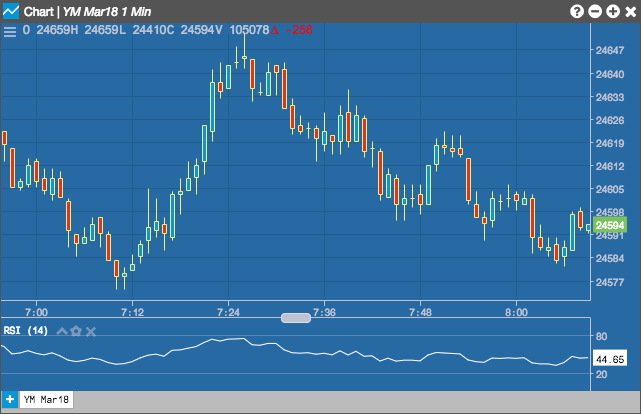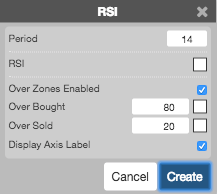Charts

# RSI

The Relative Strength Index (RSI) is used to measure underlying strength of a market move. RSI uses the prior bar's close to current bar's close change. The current price is normalized as a percentage between 0 and 100.

Relatively high RSIs (60-80) normally accompany a rising trend and relatively low RSIs (20-40) normally accompany a declining price trend. If price makes a higher high and RSI makes a lower high, the divergence between the two could signal bearish market reversals. Conversely, a lower low in price and higher low in RSI could signal a bullish market reversal.## Configuration Options• Period: Number of bars to use in the calculations.
• Color Selectors: Colors to use for graph elements.
• Over Zones Enabled: Whether to shade the area between the plot and the horizontal overbought and oversold levels.
• Over Bought: Overbought quantity
• Over Sold: Oversold quantity
• Display Axis Label: Whether to display the most recent value on the Y axis.

## Formula

$RSI = 100 - \frac{100}{(1 + RS)}$

Where:

$RS = \frac{(Average\;of\;the\;up\;closes\;over\; n\;periods)}{(Average\; of\; the\; down\; closes\; over\; n\;periods)}$

The average of the “up closes” refers to the total changes higher over the past n periods (not the last n up-periods) divided by n. The average of “down closes” refers to the total changes lower over the same span.

RSI values are smoothed in an exponential manner after the initial calculation whereby the averages of up closes and down closes are each divided by n-1 and the new period’s up or down close is added. The result is then divided by n. As follows:

$Average\; up\; close = \frac{(previous\; average\; up\; close * (n-1) + current\; up\; close)}{n}$

$Average\; down\; close = \frac{(previous\; average\; down\; close * (n-1) + current\; down\; close)}{n}$